Home > ACC7 > Chapter cc29 > Lesson cc29.2.4 > Problem9-91

9-91.
1. A bag of marbles contains only the colors red and green. Homework Help ✎

1. If the probability of getting a red marble is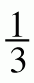, what is the probability of getting a green marble?

2. If there are 24 marbles in the bag, how many are green?

3. What is the probability of drawing two marbles that are red? Note: The first marble is not replaced before drawing the second. Write your answer as a fraction and as a percent.

4. What is the probability of drawing two red marbles if the first one is replaced before the second one is drawn? Write your answer as a fraction and as a percent.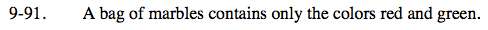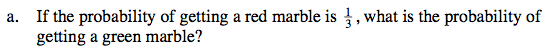The probability stated means that if there are 3 marbles in the bag, there is only one chance that you will pull out a red marble. How many chances are there that you will pull out a green marble?

If there were only three marbles in the bag, and one of them is red, then the other 2 must be green. That means that 2 out of 3 marbles are green, so the probability must be

$\frac{2}{3}.$

Another way to think of this is that if 1 of every 3 marbles is red, then the other 2 of every 3 must be green.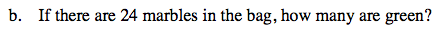If the probability of picking a green marble is two thirds, that means two thirds of the marbles are green. What is two thirds of 24?

How could you represent two thirds in a fraction with the denominator, or bottom number, of 24? The numerator, or number on top, will be the number of green marbles.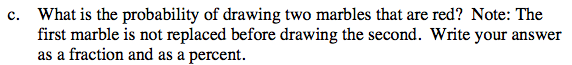To determine the probability for the first marble, use the same method as part (a). For the second marble, think of the total number of marbles as 23, because one marble has been removed. If there were 8 red marbles, and one has been removed, what number is the numerator?

To get the total probability, multiply the two probabilities for the marbles.

$\frac{7}{69}$

Can you determine the percent for this fraction?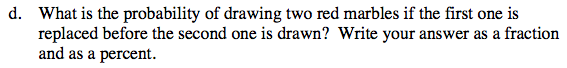Use the same method as part (c), but for the second marble, do not subtract the first marble from the fraction (numerator or denominator).

$\frac{1}{9}$

Find the probability as a percent.